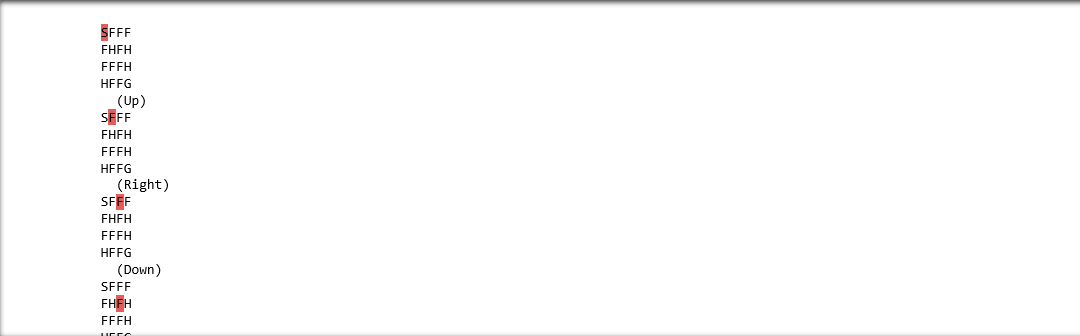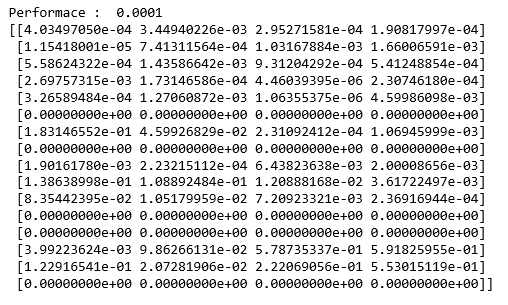# SARSA Reinforcement Learning

• Difficulty Level : Medium
• Last Updated : 24 Jun, 2021

Prerequisites: Q-Learning technique
SARSA algorithm is a slight variation of the popular Q-Learning algorithm. For a learning agent in any Reinforcement Learning algorithm it’s policy can be of two types:-

1. On Policy: In this, the learning agent learns the value function according to the current action derived from the policy currently being used.
2. Off Policy: In this, the learning agent learns the value function according to the action derived from another policy.

Q-Learning technique is an Off Policy technique and uses the greedy approach to learn the Q-value. SARSA technique, on the other hand, is an On Policy and uses the action performed by the current policy to learn the Q-value.
This difference is visible in the difference of the update statements for each technique:-

1. Q-Learning:2. SARSA:Here, the update equation for SARSA depends on the current state, current action, reward obtained, next state and next action. This observation lead to the naming of the learning technique as SARSA stands for State Action Reward State Action which symbolizes the tuple (s, a, r, s’, a’).
The following Python code demonstrates how to implement the SARSA algorithm using the OpenAI’s gym module to load the environment.
Step 1: Importing the required libraries

## Python3

 import numpy as np import gym

Step 2: Building the environment
Here, we will be using the ‘FrozenLake-v0’ environment which is preloaded into gym. You can read about the environment description here.

## Python3

 #Building the environment env = gym.make('FrozenLake-v0')

Step 3: Initializing different parameters

## Python3

 #Defining the different parameters epsilon = 0.9 total_episodes = 10000 max_steps = 100 alpha = 0.85 gamma = 0.95   #Initializing the Q-matrix Q = np.zeros((env.observation_space.n, env.action_space.n))

Step 4: Defining utility functions to be used in the learning process

## Python3

 #Function to choose the next action def choose_action(state):     action=0     if np.random.uniform(0, 1) < epsilon:         action = env.action_space.sample()     else:         action = np.argmax(Q[state, :])     return action   #Function to learn the Q-value def update(state, state2, reward, action, action2):     predict = Q[state, action]     target = reward + gamma * Q[state2, action2]     Q[state, action] = Q[state, action] + alpha * (target - predict)

Step 5: Training the learning agent

## Python3

 #Initializing the reward reward=0   # Starting the SARSA learning for episode in range(total_episodes):     t = 0     state1 = env.reset()     action1 = choose_action(state1)       while t < max_steps:         #Visualizing the training         env.render()                   #Getting the next state         state2, reward, done, info = env.step(action1)           #Choosing the next action         action2 = choose_action(state2)                   #Learning the Q-value         update(state1, state2, reward, action1, action2)           state1 = state2         action1 = action2                   #Updating the respective vaLues         t += 1         reward += 1                   #If at the end of learning process         if done:             breakIn the above output, the red mark determines the current position of the agent in the environment while the direction given in brackets gives the direction of movement that the agent will make next. Note that the agent stays at it’s position if goes out of bounds.
Step 6: Evaluating the performance

## Python3

 #Evaluating the performance print ("Performance : ", reward/total_episodes)   #Visualizing the Q-matrix print(Q)My Personal Notes arrow_drop_up
Recommended Articles
Page :